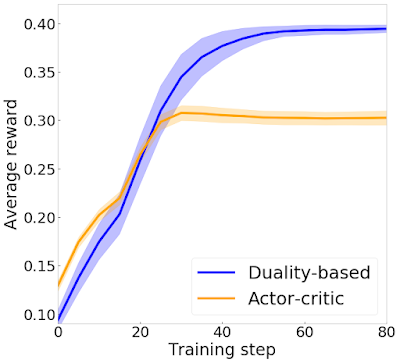# Duality — A New Approach to Reinforcement Learning

Posted by Ofir Nachum and Bo Dai, Research Scientists, Google Research

Reinforcement learning (RL) is an approach commonly used to train agents to make sequences of decisions that will be successful in complex environments, including for example, settings such as robotic navigation, where an agent controls the joint motors of a robot to seek a path to a target location, or game-playing, where the goal might be to solve a game level in minimal time. Many modern successful RL algorithms, such as Q-learning and actor-critic, propose to reduce the RL problem to a constraint-satisfaction problem, where a constraint exists for every possible “state” of the environment. For example, in vision-based robotic navigation, the “states” of the environment correspond to every possible camera input.

Despite how ubiquitous the constraint-satisfaction approach is in practice, this strategy is often difficult to reconcile with the complexity of real-world settings. In practical scenarios (like the robotic navigation example) the space of states is large, sometimes even uncountable, so how can one learn to satisfy the tremendous number of constraints associated with arbitrary input? Implementations of Q-learning and actor-critic often ignore these mathematical issues or obscure them through a series of rough approximations, which results in a stark divide between the practical implementations of these algorithms and their mathematical foundations.

In “Reinforcement Learning via Fenchel-Rockafellar Duality” we have developed a new approach to RL that enables algorithms that are both useful in practice and mathematically principled — that is to say, the proposed algorithms avoid the use of exceedingly rough approximations to translate their mathematical foundations to practical implementation. This approach is based on convex duality, which is a well-studied mathematical tool used to transform problems expressed in one form into equivalent problems in distinct forms that may be more computationally friendly. In our case, we develop specific ways to apply duality in RL to transform the traditional constraint-satisfaction mathematical form to an unconstrained, and thus more practical, mathematical problem.

A Duality-Based Solution
The duality-based approach begins by formulating the reinforcement learning problem as a mathematical objective along with a number of constraints, potentially infinite in number. Applying duality to this mathematical problem yields a different formulation of the same problem. Still, this dual formulation has the same format as the original problem — a single objective with a large number of constraints — although the specific objective and constraints are changed.

The next step is key to the duality-based solution. We augment the dual objective with a convex regularizer, a method often used in optimization as a way to smooth a problem and make it easier to solve. The choice of the regularizer is crucial to the final step, in which we apply duality once again to yield another formulation of an equivalent problem. In our case, we use the f-divergence regularizer, which results in a final formulation that is now unconstrained. Although there exist other choices of convex regularizers, regularization via the f-divergence is uniquely desirable for yielding an unconstrained problem that is especially amenable to optimization in practical and real-world settings which require off-policy or offline learning.

Notably in many cases, the applications of duality and regularization prescribed by the duality-based approach do not change the optimality of the original solution. In other words, although the form of the problem has changed, the solution has not. This way, the result obtained with the new formulation is the same result as for the original problem, albeit achieved in a much easier way.

Experimental Evaluation
As a test of our new approach, we implemented duality-based training on a navigational agent. The agent starts at one corner of a multi-room map and must navigate to the opposite corner. We compare our algorithm to an actor-critic approach. Although both of these algorithms are based on the same underlying mathematical problem, actor-critic uses a number of approximations due to the infeasibility of satisfying the large number of constraints. In contrast, our algorithm is more amenable to practical implementation as can be seen by comparing the performance of the two algorithms. In the figure below, we plot the average reward achieved by the learned agent against the number of iterations of training for each algorithm. The duality-based implementation achieves significantly higher reward compared to actor-critic.A plot of the average reward achieved by an agent using the duality-based approach (blue) compared to an agent using standard actor-critic (orange). In addition to being more mathematically principled, our approach also yields better practical results.

Conclusion
In summary, we’ve shown that if one formulates the RL problem as a mathematical objective with constraints, then repeated applications of convex duality in conjunction with a cleverly chosen convex regularizer yield an equivalent problem without constraints. The resulting unconstrained problem is easy to implement in practice and applicable in a wide range of settings. We’ve already applied our general framework to agent behavior policy optimization as well as policy evaluation, and imitation learning. We’ve found that our algorithms are not only more mathematically principled than existing RL methods, but they also often yield better practical performance, showing the value of unifying mathematical principles with practical implementation.Read More